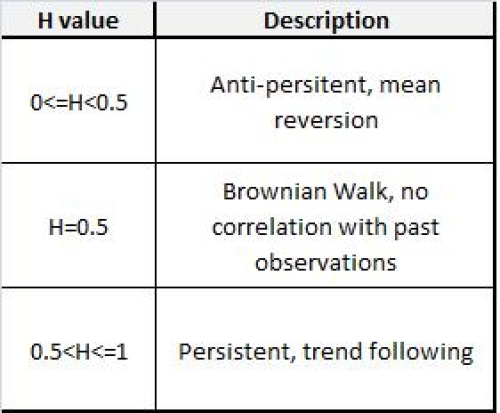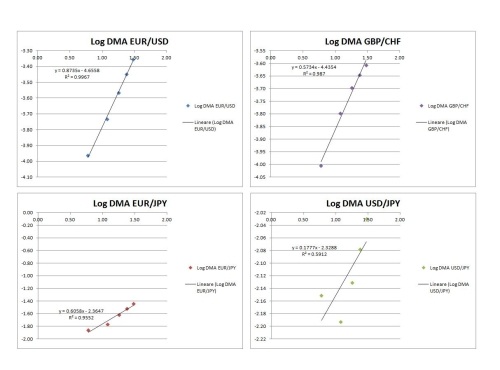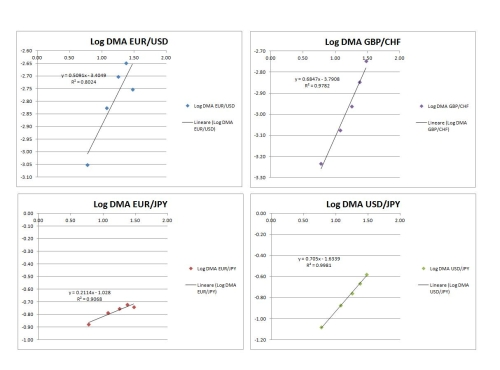Abstract

In the following work I will introduce the Hurst Exponent and some briefexplanation of the known methodologies of estimation. The estimations made on this work will apply to closing prices on different time frames and pair crosses, using exclusively the Detrending Moving Averageanalysis, which from here on will be indicated as DMA.

Introduction

The Hurst Exponent is applied in a vast range of disciplines, fromhydrology, where it was first introduced, to computer networks, medicine, biology, and of course: Finanace. The parameter was first introduced in 1951 by Harold Hurst (1880-1978), a British Hydrologist who, at that time, was in charge of determining the optimum dam sizing of the Nile river. Yearly observations on the levels of the Nile river where available, covering a fairly long period of time in which consecutive observations seemed to be correlated, showing a long-term memory. Hurst implemented a new statistical method to estimate the long term memory: The Rescaled Range Analysis, widely described in his book.

The Hurst exponent (H) can only assume values between 0 a 1. The following table shows how to interpret the different values of H.Estimation Methodologies of the Hurst Exponent

There are many techniques to estimate the Hurst Exponent, bear in mind thatall of these techniques estimate the parameter but none of them measures the true value.

Some of the methodologies availabe are:
• Rescaled Range Analysis (RRA)
• Detrended Fluctuation Analysis (DFA)
• Wavelet-based Estimation
• Geometrical Method (GM)
• Detrending Moving Average (DMA)
I haven't test them all, just a few of them, the DFA and DMA are verysimilar in terms of calculations and results, while the RRA has some problems overstimating H, sometimes going beyond its upper limit: 1, although some corrections are available on academic papers.

Detrending Moving Average

The function of the DMA is the following:Where:

• y(t) is the value of the time series with index t;
• yn(t) is the moving average with period n (sorry I couldn't insert the tilde on y);
• n is the period of the moving average;
• Nmax is the window's size for calculation.
Steps to calculate sigma DMA:
2. Choose the the size of the window, the authors recommend a number betwen 2000 : 3000 observations, the DMA analysis will be applied to these observations;
3. Choose the different periods for the moving averages, for example: start with nstart = 10 with a step of nstep = 10 and a final value of nend= 1000;
4. For the first value outside the window you will get different sigma DMA values for each n, plot them as a function of n in
a log-log axes;
5. Perform a linear regression on the series to obtain an equation in which the slope represents the Hurst coefficient.
DMA Algorithm Applied

Here are some graphic results of the process described above, taking intoaccount the following parameters:
• Time Series: Close Prices, chosen on random dates
• n: start(6), step(6), end(30)
• Nmax: 60
Calculation paramaters are not the ones suggested by the authors, but lackof time and a spreadsheet as my only sidekick didn't help much.

1 minute TF15 minutes TF1 hour TF1 day TFConclusions

The DMA analysis seems to perform well using closing prices as the time series, usually the calculations are performed on the returns. Most of the R squared are fairly close to 1, showing a good fitting. Further and more complex calculations need to be done in order to see if this method is of any use in technical analysis.

References

1- Second-order moving averages and scaling of stochastic time series (E. Alessio ,A.Carbone, G. Castelli and V. Frappietro, 2002)
2- Long-Term Storage: An ExperimentalStudy (Hurst et al., 1965)
3- Some comments on Hurst exponent and the long memory processes on capital markets ( M.A. Sánchez Granero, J.E. Trinidad Segovia, J. García Pérez, 2008)
Translate to English Show original